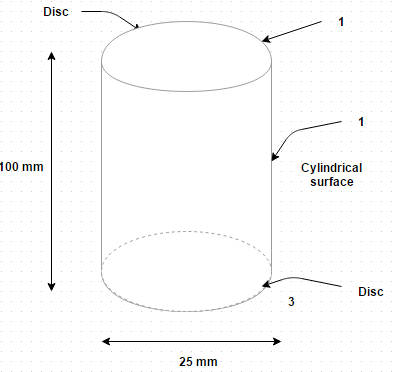Courses

## 10 Questions MCQ Test Heat Transfer | Test: Gaseous Radiations

Description
This mock test of Test: Gaseous Radiations for Chemical Engineering helps you for every Chemical Engineering entrance exam. This contains 10 Multiple Choice Questions for Chemical Engineering Test: Gaseous Radiations (mcq) to study with solutions a complete question bank. The solved questions answers in this Test: Gaseous Radiations quiz give you a good mix of easy questions and tough questions. Chemical Engineering students definitely take this Test: Gaseous Radiations exercise for a better result in the exam. You can find other Test: Gaseous Radiations extra questions, long questions & short questions for Chemical Engineering on EduRev as well by searching above.
QUESTION: 1

### Which of the following have a continuous spectrum?

Solution:

They emit and absorb radiant energy of all wavelength.

QUESTION: 2

### Which of the following is/are known as selective absorbers?

Solution:

They emit and absorb radiant energy in definite parts of the spectrum called bands.

QUESTION: 3

### Which layers participated in the process of thermal radiation through solids and liquids?

Solution:

Gases possess a much small radiating power and all their volumes participate in the radiation.

QUESTION: 4

Some of the gases have low emissive power and absorptivity. They are considered practically diathermanous. Which of the following option is true?

Solution:

Helium is monoatomic gas and is extremely inert to thermal radiation.

QUESTION: 5

Consider an enclosure formed by three surfaces having the following values of shape factors, emissivities and temperaturesSurface 1 i.e. curved cylindrical has an emissivity 0.75 and temperature 800 K
Surface 2 i.e. closing disc has an emissivity 0.8 and temperature 700 K
Surface 3 i.e. closing disc has an emissivity 0.8 and temperature 700 K
The closing flat discs are 25 mm in diameter and they have interspacing distance equal to 100 mm. If the shape factor between these two identical discs is 0.05, calculate the net rate of radiant heat flow from the curve surface to each of the closing end surface

Solution:

F 21 + F 23 + 1 and A = 0.00785 m2, A= 0.000491 m2. Then from reciprocity theorem, F 12 = 0.0594. Q12 = F 12 σ (T 1– T 2 4) = 3.561 W.

QUESTION: 6

Consider a beam of monochromatic radiation at wavelength λ that enters a layer of absorbing gas. As the beam passes through the gas layer, its intensity

Solution:

The decrease is given by d I λ X = – K λ λ X d x.

QUESTION: 7

When a gas or vapor is in the process of oxidation and combustion, it is called as

Solution:

It is known as flame.

QUESTION: 8

The monochromatic absorption coefficient depends upon
(i) Temperature
(ii) Time
(iii) Pressure
(iv) Wavelength
Identify the correct option

Solution:

It depends upon the state of gas and the wavelength.

QUESTION: 9

Which one of the following is not extremely inert to thermal radiation?

Solution:

Methane is a polyatomic gas.

QUESTION: 10

Consider a beam of monochromatic radiation at wavelength λ that enters a layer of absorbing gas. As the beam passes through the gas layer, its intensity is given by

Solution:

Its intensity gets reduced.